Example: Bearing structure with a machine
Problem description:Supporting machines on structures is an important task in the field of machine dynamics. Besides the dynamic behaviour of the machine, it is also important to consider the dynamics of the supporting structure, specially at the mounting points. The vibrations transmitted into the base structure can be reduced using elastic mounts. In this example an internal combustion engine is placed on a structure representing a section of a ship (see Figure 1). It is possible to reduce the transmission of vibration by optimizing the mounting elements or by designing additional vibration control measures.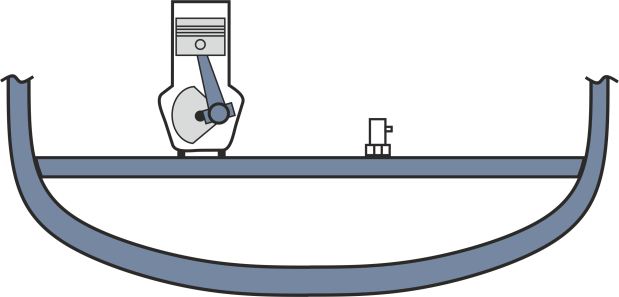Figure 1: Internal combustion engine in a ship

Simplification of the stucture:
For a first investigation the machine motor is considered as rigidly fixed on the elastic support structure. Based on this initial model the influence of the machine-vibrations on the base structure is estimated and possible passive vibration reduction methods are evaluated and discussed. Real structures often show a high level of complexity. However, for an initial investigation it is often useful to reduce the level of complexity and to simplify the model. In our case we simplify the supporting structure as a simply supported beam with an E- Young’s modulus E, the area moment of inertia I and density per unit length µ (see Figure 2). The motor is idealized as an unbalanced mass exciter with a total mass m, a rotational frequency Ω and a unbalanced mass mu, which is rotating around a radius of r. The resulting imbalance force is defined by F(t)=mu2sin(Ωt).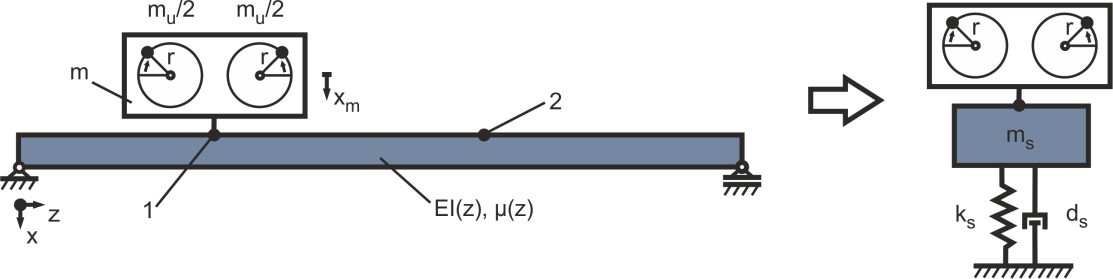Figure 2: Simplification of the structure as simply supported beam and the equivalent mass-spring-system with one degree of freedom

The beam cross section is considered to be that of an I-Profile with a wall thickness t = 50 mm, a height h = 300 mm and a total length of 1200 mm (see Figure 3). The beam material is steel with a Young’s modulus E = 210 kN/mm² and a material density of ρ = 7850 kg/m³. Based on this information the moment of inertia can be calculated to be I = t*h³/2 = 1.6e9 mm4 . Considering the damping as sufficiently small the first three natural frequencies can be calculated with f_n=(nπ^2)/2π √(EI/(µl^4 )) as f1 = 29.14 Hz, f2 = 116.54 Hz and f3 = 262.21 Hz, where the length dependent-mass is approximated to be µ=3ρht=471 kg/m. The corresponding modeshapes a1, a2 and a3 are depicted in Figure 3.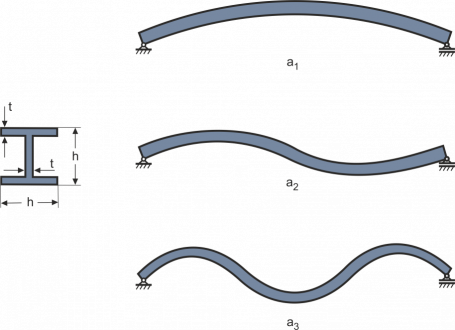Figure 3: Dimensions of the I-profile and the first three eigenforms

As machine a common 12 cylinder combustion engine with an overall weight of 600 kg is considered. The cylinders have a weight of 16 kg each with a stoke of 190 mm. The typical frequency range of this motor is between 13 – 33 Hz corresponding to a revolution speed of 800 – 2000 rpm. This means that first natural frequency of the supporting structure at 29,14Hz is of special interest,since it lays inside the operational frequecy range of the engine. For the subsequent simulations the structure is considered as a damped harmonic oscillator system with the characteristics (natural frequency, modal mass and stiffness) of the first eigenmode. This way the model of the structure has been reduced to a mass ms, a stiffness ks and a damper ds (see Figure 2). As a further simplification the location of the force excitation 1 and the observation point 2 are considered to be collocated in the middle of the beam at z = l/2. For the first modeshape the equivalent mass can be calculated using the energy method to ms=∫µψ^2 dz. With the sinusoidal eigenvector ψ=sin[πz/L] follows ms=(µL)/2=282.6 kg . For first natural frequency the equivalent stiffness can be calculated to be ks=9470328 N/m. For the damping the structural a damping coefficient of ds = 2000 kg/s is considered, which results in 4% modal damping.

Table 1: Parameter of the simulation

 Parameter Value Thickness of the beam 0.05 m Height of the beam 0.4 m Length of the beam 1.2 m Structural damping 2000 kg/s Young’s modulus 210 kN/mm² Density 7850 kg/m³ Engine mass 600 kg Cylinder mass 16 kg Cylinder stroke 190 mm Revolution speed 800 – 2000 rpm Simulation sampling time 1e-3 s Simulation time 30 s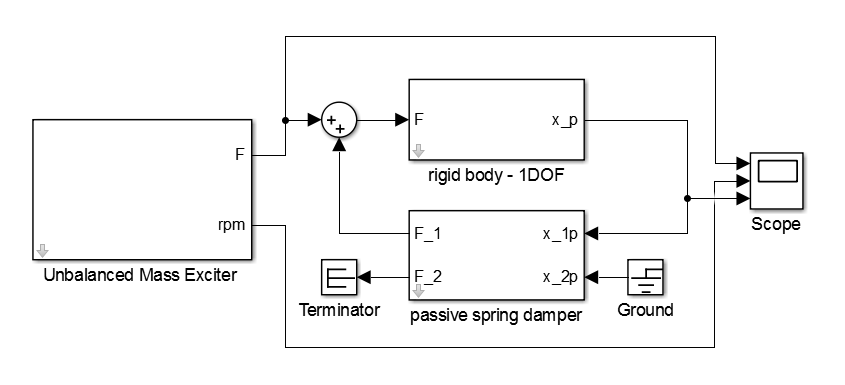Figure 4: Simulink® block-diagram of the damped harmonic oscillator

For time-domain simulations the Simulink® model shown in figure 4 is implemented.The model uses a fixed-step solver and a fundamental simulation sample time of 1e-3 s. The simulation time period is 30 seconds. For the rigid mass the passive spring damper and the excitation the respective blocks are taken from AdaptroSimTM Structure and Vibration toolbox and parametrized using the previous derived parameters. For the unbalanced mass exciter an excitation range from 0 to 3000 rpm with a run up speed of 100 1/min/s is defined.

Results:
The time-domain results are shown in Figure 5. Here the excitation force and the rpm of the unbalanced mass exciter and the displacement of the systems mass can be depicted.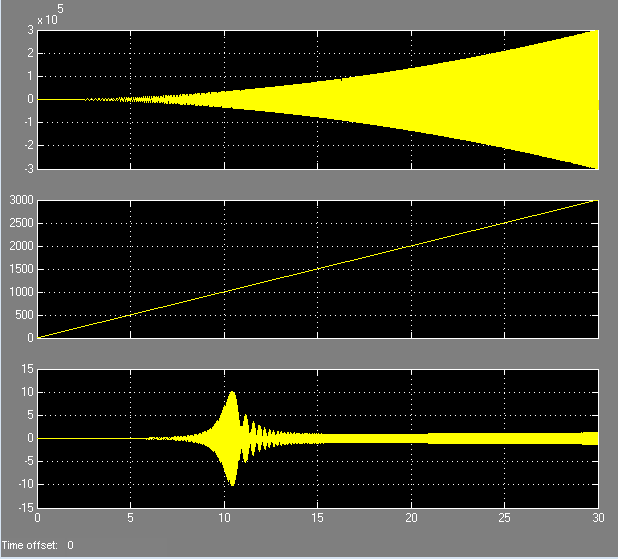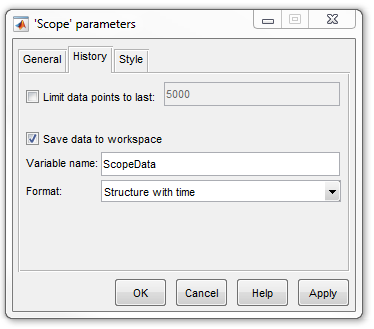Figure 5: a) Scope of the Simulink® model and b) Setup for data export

For an evaluation in the frequency domain the time data is exported to MATLAB® for a post-processing. To do this the Scope-block(See figure 5) is modified to save the data to the MATLAB® workspace. The data is processed with the ma_frequency_response function.

ma_frequency_response(ScopeData.time, ScopeData.signals(1).values, ScopeData.signals(3).values,{0,50})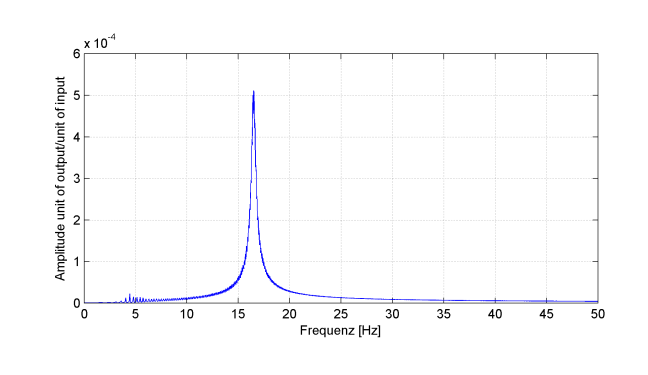Figure 6: Frequency domain resulsts from post-processing in MATLAB®

The frequency domain results for the amplitude of the frequency response function between the displacement of the system mass and the excitation force are shown in Figure 6. The system response shows a high peak around the system resonance frequency, which occurs at 16.5 Hz . This frequency results from the ridged coupling of the exciter with its total mass m on the beam. This result is in good agreement with the analytical result of the natural frequency of the 1DOF-system f=√(k/m)/(2π)=16.48 Hz.